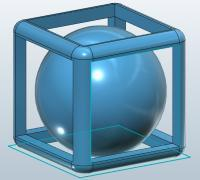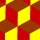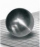# Inscribed sphere

How many % of the volume of the cube whose edge is 6 meters long is a volume of a sphere inscribed in that cube?

p =  52.3599 %

### Step-by-step explanation:Did you find an error or inaccuracy? Feel free to write us. Thank you!Tips to related online calculators
Check out our ratio calculator.
Tip: Our volume units converter will help you with the conversion of volume units.

#### You need to know the following knowledge to solve this word math problem:

We encourage you to watch this tutorial video on this math problem:

## Related math problems and questions:

• Inscribed sphereHow many percent of the cube volume takes the sphere inscribed into it?
• Cube in sphereThe cube is inscribed in a sphere with a radius r = 6 cm. What percentage is the volume of the cube from the volume of the ball?
• Sphere vs cubeHow many % of the surface of a sphere of radius 12 cm is the surface of a cube inscribed in this sphere?
• Cube in ballCube is inscribed into the sphere of radius 181 dm. How many percent is the volume of cube of the volume of the sphere?
• Reduce of the volumeCalculate how many % reduce the volume of the cube is we reduced length of each edge by 10%.
• Cube zoomHow many percents do we increase the volume and surface of the cube if we magnify its edge by 38 %?
• The cubeThe cube has an edge of 12 dm. The second cube has an edge exactly 20% longer. How many % is more water in the second cube than in the first cube, if the first cube is full to 3/4 and the second to 3/8?
• InscribedCube is inscribed in the cube. Determine its volume if the edge of the cube is 10 cm long.
• Cube in sphereThe sphere is an inscribed cube with an edge of 8 cm. Find the sphere's radius.
• Cube cutIn the ABCDA'B'C'D'cube, it is guided by the edge of the CC' a plane witch dividing the cube into two perpendicular four-sided and triangular prisms, whose volumes are 3:2. Determine in which ratio the edge AB is divided by this plane.
• Cube edgesIf the edge length of the cube increases by 50%, how does the volume of this cube increase?
• Cube, cuboid, and sphereVolumes of a cube and a cuboid are in ratio 3: 2. Volumes of sphere and cuboid are in ratio 1: 3. At what rate are the volumes of cube, cuboid, and sphere?
• Cube into sphereThe cube has brushed a sphere as large as possible. Determine how much percent was the waste.
• LatheCalculate the percentage of waste if the cube with 53 cm long edge is lathed to cylinder with a maximum volume.The radius of the sphere we reduce by 1/3 of the original radius. How much percent does the volume and surface of the sphere change?A cube with an edge 1 m long is a circumscribed sphere (vertices of the cube lie on a sphere's surface). Find the surface area of the sphere.How many percent volume remain of a sphere if diameter decrease 3×?